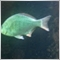# Indicators14

Hello, I'm new to mql5 so please bear with me.

I found an indicator here on codebase that I like but to call it into an ea I need to know the parameters. I don't know how to find that. Can someone help me?

Here's the indicator:

```//+------------------------------------------------------------------+
//|                                       Support and Resistance.mq5 |
//|                                       Update Dec 2014            |
//+------------------------------------------------------------------+
//---- version
#property version   "1.01"
//---- indicator in the chart window
#property indicator_chart_window
//---- 2 indicator buffers are used
#property indicator_buffers 2
//---- 2 graphic plots are used
#property indicator_plots   2
//+----------------------------------------------+
//|  Bearish indicator options                   |
//+----------------------------------------------+
//---- drawing type as arrow
#property indicator_type1   DRAW_ARROW
//---- Magenta color
#property indicator_color1  Magenta
//---- Line width
#property indicator_width1  1
//---- Support label
#property indicator_label1  "Support"
//+----------------------------------------------+
//|  Bullish indicator options                   |
//+----------------------------------------------+
//---- drawing type as arrow
#property indicator_type2   DRAW_ARROW
//---- Lime color
#property indicator_color2  Lime
//---- Line width
#property indicator_width2  1
//---- Resistance label
#property indicator_label2 "Resistance"

//+----------------------------------------------+
//| Indicator input parameters                   |
//+----------------------------------------------+
//input int iPeriod=70; // ATR period
//+----------------------------------------------+

//---- declaration of dynamic arrays, used as indicator buffers
double SellBuffer[];
//---
int StartBars;
int FRA_Handle;
//+------------------------------------------------------------------+
//| Custom indicator initialization function                         |
//+------------------------------------------------------------------+
void OnInit()
{
//---- initialization of global variables
StartBars=6;
//---- get handle of the iFractals indicator
FRA_Handle=iFractals(NULL,0);
if(FRA_Handle==INVALID_HANDLE)Print(" INVALID_HANDLE FRA");

//---- set SellBuffer as indicator buffer
SetIndexBuffer(0,SellBuffer,INDICATOR_DATA);
//---- set indxex of starting bar to plot
PlotIndexSetInteger(0,PLOT_DRAW_BEGIN,StartBars);
//---- set label for support
PlotIndexSetString(0,PLOT_LABEL,"Support");
//---- set arrow char code
PlotIndexSetInteger(0,PLOT_ARROW,159);
//---- set indexing as timeseries
ArraySetAsSeries(SellBuffer,true);

//---- set BuyBuffer as an indicator buffer
//---- set index of starting bar to plot
PlotIndexSetInteger(1,PLOT_DRAW_BEGIN,StartBars);
//---  set label for resistance
PlotIndexSetString(1,PLOT_LABEL,"Resistance");
//---- set arrow char code
PlotIndexSetInteger(1,PLOT_ARROW,159);
//---- set indexation as timeseries

//---- set precision
IndicatorSetInteger(INDICATOR_DIGITS,_Digits);
//---- indicator short name
string short_name="Support & Resistance";
IndicatorSetString(INDICATOR_SHORTNAME,short_name);
//----
}
//+------------------------------------------------------------------+
//| Custom indicator iteration function                              |
//+------------------------------------------------------------------+
int OnCalculate(const int rates_total,
const int prev_calculated,
const datetime &time[],
const double &open[],
const double &high[],
const double &low[],
const double &close[],
const long &tick_volume[],
const long &volume[],
)
{
//---- checking of bars
if(BarsCalculated(FRA_Handle)<rates_total
|| rates_total<StartBars)
return(0);

//---- declaration of local variables
int to_copy,limit,bar;
double FRAUp[],FRALo[];

//---- calculation of bars to copy
//---- and starting index (limit) for bars recalculation loop
if(prev_calculated>rates_total || prev_calculated<=0)// checking the first call
{
to_copy=rates_total;           // bars to copy
limit=rates_total-StartBars-1; // starting index
}
else
{
to_copy=rates_total-prev_calculated+3; // bars to copy
limit=rates_total-prev_calculated+2;   // starting index
}

//---- set indexing as timeseries
ArraySetAsSeries(FRAUp,true);
ArraySetAsSeries(FRALo,true);
ArraySetAsSeries(high,true);
ArraySetAsSeries(low,true);

//---- copy indicator data to arrays
if(CopyBuffer(FRA_Handle,0,0,to_copy,FRAUp)<=0) return(0);
if(CopyBuffer(FRA_Handle,1,0,to_copy,FRALo)<=0) return(0);

//---- main loop
for(bar=limit; bar>=0; bar--)
{
SellBuffer[bar] = 0.0;

if(FRALo[bar] != DBL_MAX) SellBuffer[bar] = low[bar]; else SellBuffer[bar] = SellBuffer[bar+1];
}
//----
return(rates_total);
}
//+------------------------------------------------------------------+

```36577

Tim G.:

Hello, I'm new to mql5 so please bear with me.

I found an indicator here on codebase that I like but to call it into an ea I need to know the parameters. I don't know how to find that. Can someone help me?

Here's the indicator:

Hello,

this indicator has not input parameters to call from expert's code.

Just use only the name of indicator on iCustom().